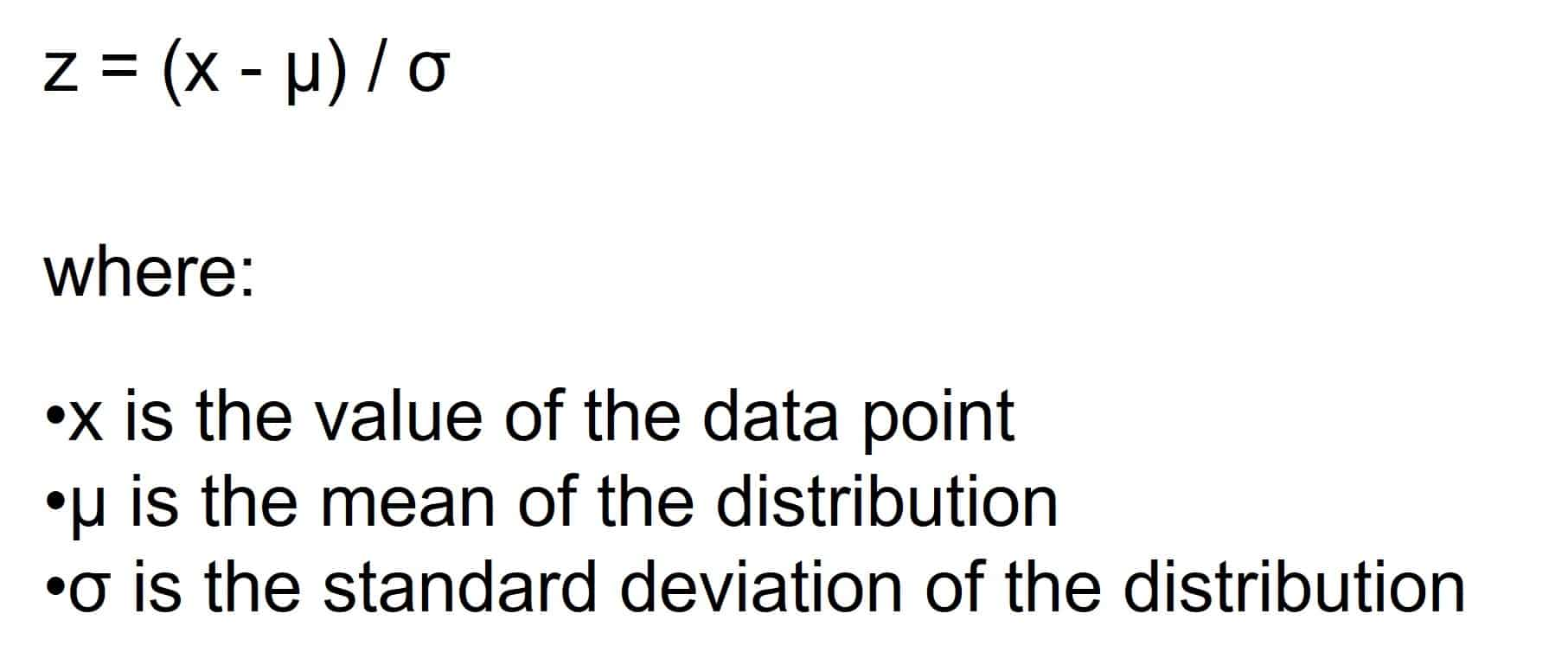Some say that Z is the sign of Zorro (Spanish for fox), the fictional sword wielding masked vigilante who defended and helped the poor. In statistics, it is something different. In statistics, z usually refers to the standardized score or z-score of a data point in a normal distribution.

The z-score measures the number of standard deviations that a data point is above or below the mean of the distribution. It is calculated as:The z-score can be used to compare values from different normal distributions, as it expresses each value in terms of its distance from the mean in units of standard deviation. It is also useful in identifying outliers or extreme values in a data set.

## Overview: What is Z?

The z-score, or standardized score, is a useful statistical tool in many ways. Some of the benefits of using the z-score include:

### Standardization

The z-score standardizes the data by converting it into a common scale. This allows for easy comparison between different datasets that have different means and standard deviations.

### Normal distribution

The z-score assumes that the data is normally distributed. This allows for the use of statistical tests that rely on the normal distribution, such as hypothesis testing and estimations of confidence intervals.

### Outlier detection

The z-score is used to identify outliers in a dataset. Any data point with a z-score greater than 3 or less than -3 is considered an outlier.

### Probability calculations

The z-score can be used to calculate probabilities and percentiles for a given dataset. This is particularly useful in hypothesis testing, where you can calculate the probability of observing a given result by chance.

### Data transformation

The z-score can be used to transform a dataset into a standard normal distribution. This transformation can be useful in data analysis, as it simplifies the calculation of certain statistics and allows for easier interpretation of results.

## An industry example of Z

Let’s assume you are analyzing the sales performance of a team of salespeople. The mean sales of the group are \$75,000, and the standard deviation is \$10,000. You want to know how well a particular salesperson is performing relative to the rest of the team if their sales are \$85,000.

To find out, you can calculate the z-score as:

z = (x – mu) / s.d    where  z = (85,000 – 75,000) / 10,000    or  z = 1.

This means that the salesperson’s score is 1 standard deviation above the mean of the distribution. Since the standard deviation is \$10,000, this translates to sales of \$85,000 being \$10,000 dollars above the mean sales of the group.

You can interpret the z-score as follows: the salesperson’s sales is better than the sales of 84.13% of the sales team assuming a normal distribution. This can be found by looking up the z-score in a standard normal distribution table or by using statistical software.

### What is a z-score?

A z-score is a standardized score that measures the number of standard deviations a data point is away from the mean of a normal distribution.

### What does a positive z-score mean?

A positive z-score means that a data point is above the mean of the distribution.

### What does a negative z-score mean?

A negative z-score means that a data point is below the mean of the distribution.

### How is the z-score calculated?

The z-score is calculated by subtracting the mean of the distribution from the data point and then dividing the result by the standard deviation of the distribution.

### What is the purpose of the z-score?

The z-score is used to standardize data and compare values from different normal distributions. It is also used to identify outliers and calculate probabilities and percentiles.

### What is the range of z-scores?

The range of z-scores is from -infinity to +infinity. However, in practice, most z-scores fall within the range of -3 to +3. This will encompass 99.73% of the data points for a normal distribution.

### What is a good z-score?

A good z-score depends on the context and the specific application. In general, a z-score of 0 means that a data point is equal to the mean of the distribution, while a z-score of 1 indicates that a data point is 1 standard deviation above the mean. A z-score of 2 or 3 is considered high and may indicate an outlier.

### Can the z-score be negative?

Yes, the z-score can be negative if a data point is below the mean of the distribution.

### Is the z-score affected by outliers?

Yes, the z-score can be affected by outliers, especially if they are extreme. In such cases, it may be necessary to use alternative methods to deal with outliers.

### Can the z-score be used for non-normal distributions?

No, the z-score assumes a normal distribution and may not be appropriate for non-normal distributions. In such cases, alternative methods should be used.